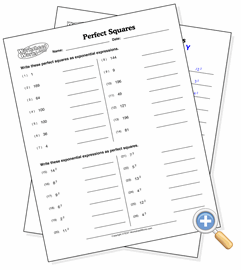# Perfect SquaresRecognize and calculate the roots of squares

The Perfect Squares worksheet presents the student with a list of numbers that are the square of an integer. The student’s task is to figure out what that integer is.

The typical way to accomplish this is to estimate what the root might be and then try squaring it to see if it matches the square. On the easiest level, the squares are values that are beneficial to memorize. On the larger levels, guessing at roots gives the student practice in eyeballing roots of numbers, a good skill to have in algebra and calculus.